• 利用matplotlib绘制函数图像
千次阅读
2018-09-19 21:15:53

### 主要步骤：

准备数据
配置画板
绘制
保存
代码如下：

import numpy as np
import matplotlib.pyplot as pt
from matplotlib import font_manager

# pt.rcParams["font.sans-serif"] = ['SimHei']
# 设置中文字体显示
my_font = font_manager.FontProperties(fname="C:\Windows\Fonts\simsun.ttc")
# 准备数据
X = np.linspace(-np.pi, np.pi * 2, 1000)
# 配置画板，figsize用来设置图例的大小
pt.figure(figsize=(6,3),facecolor="yellow")
# subplot的第一个参数表示行，第二个参数表示列，第三个参数表示图例中的激活位置
pt.subplot(1,1,1)
# 准备Y数据
Y_x2 = X1**2
Y_sin = np.sin(X)

# 标题，X坐标和Y坐标名称
pt.xlabel(u"X数值",fontproperties=my_font)
pt.ylabel(u"Y数值",fontproperties=my_font)
pt.title(u"函数图像",fontproperties=my_font,fontsize=20)

# 设置X,Y轴上下限
pt.xlim(-np.pi,np.pi)
pt.ylim(-1,1)

# 设置关键刻度
pt.xticks([-np.pi,-np.pi/2.0,0,np.pi/2,np.pi])
# 添加文字，第一个参数为X坐标，第二个参数是Y轴坐标，以数据的刻度为基准
pt.text(0.0,-0.5,'dons move',fontdict={'size':'16','color':'b'})
# 添加标注，xy是标注箭头要指的点，xytext：描述信息的坐标，arrowprops箭头的样式
pt.annotate('note!!',xy=(-np.pi/2.0,-1),xytext=(-np.pi/2,-0.25),fontsize=16,arrowprops=dict(facecolor='black',shrink=0.01))

# 保存到文件
pt.savefig("test2.jpg")

# 图例
pt.plot(X1, Y_x2,c="g",lw=3,label=u"x2函数")
# 直线-，虚线--，点线:，点划线-.
pt.plot(X, Y_sin,c="r",lw=5,ls="-.",label=u"sin函数")
# 添加图例
pt.legend(prop=my_font)

pt.show()

更多相关内容
• 通过matplotlib绘制自己不会画的高数的图像，方便观察、解题。

很多考研同学此时此刻都在为了高数而头疼，很多同学看到函数之后想利用函数图像来判断某些问题，方便明了而且简洁的利用图像来解决问题。但是在观察图形之前有一个很令人头疼的问题：我们此时此刻遇到的函数已经不是简单的y=x+1了，动辄就是各种二次三次加求导，图都画不出来又谈何观察答案呢。那么我们就用到了python的matplotlib库，Matplotlib 是一个非常强大的 Python 画图工具，我们可以使用该工具将很多数据通过图表的形式更直观的呈现出来。可以绘制线图、散点图、等高线图、条形图、柱状图、3D 图形、甚至是图形动画等等。那么它同样可以来解决我们的函数图像问题。

那么我们开始来着手实际的操作一下：

1. 导入科学计算包Numpy和快速绘图模块pyplot，其中Numpy是matplotlib库的基础，即matplotlib是建立在Numpy基础之上的Python会图库

这里推荐拿清华大学开源软件镜像站下载，速度快且方便，用了都说好。

打开terminal后：

pip install -i Simple Index matplotlib

2、接下来我们来导入包并且重命名。

import matplotlib.pyplot as plt
import numpy as np

3、下一步我们要来确定坐标轴的长度以及刻度

# 确定坐标轴
plt.xlim((-3, 3))
plt.ylim((-70, 150))

Xlim和ylim都是用来写x，y刻度的函数，里面的数字可以换成自己函数的定义域。

4、前置工作准备好之后我们就可以开始写自己的函数了。

# 确定函数的x,y点

# 原函数
x1 = np.linspace(-3, 3.5, 100)

def y1(x1):
y1 = 3 * x1 ** 3 + 2 * x1 ** 2 + x1 + 4
return y1


这里的linspace函数是干什么用的呢？

Linspace函数：x=np.linspace(start，end，portion)。这里又有小伙伴会问了那么这个start，end，portion分别是什么意思呢？这里指函数中的三个参数，start代表起始值，end代表结束值，portion代表分割的份数。那么在图示中所示的三个参数意味把从-3到3.5之间的部分分为100份。

接下来定义y，这里就不细说了，只是简单的函数方程而已，a*b为a×b，a**b为a^b.

如果想的话可以写一下他的导函数，方便进一步观察。

# 一阶导数
x2 = x1

def y2(x2):
y2 = 9 * x2 ** 2 + 4 * x2 + x2
return y2

# 二阶导数
x3 = x1

def y3(x3):
y3 = 18 * x3 + 4
return y3


5、这里我们写完了函数就到了生成图像的时候了。生成图像我们要用到plot函数。

# plot为绘制图像的函数，label为标记
plt.plot(x1, y1(x1), 'r-', linewidth=1, label='f(x)')
plt.plot(x2, y2(x2), 'g--', linewidth=1, label="f '(x)")
plt.plot(x3, y3(x3), 'b-', linewidth=0.8, label="f ' '(x)")

Plot函数中第一个参数是x坐标，第二个参数为y轴坐标。之后的’r-’指的是这个函数生成的图像线条颜色会是红色的“-”线，如果写成’r--’，图像就会是红色的“--”虚线。其他两个同理。这里可以根据个人爱好自己改一改。Linewidth为图像线条的宽图（如果为了观察函数交点，那就不要设的太宽，要不然选错了题那就是：“经典的错误，标准的零分”。Label为标记，不会对结果有什么影响，也不会影响观看，因为我们后面会写图例。

6、接下来我们给这一条函数写一条注释：

# 在图上添加文字注释
plt.text(-2.8, y1(3), 'f(x)=3x**3 + 2x**2 + x + 4', size=13)

Text内第一个参数和第二个参数是显示的位置（显示在以图片内横纵坐标为坐标参数的地方），可以根据自己的情况调一调。（ps：如果改了数值之后发现图片上没有，那就说明坐标改的太过导致显示出了界面，并不是bug，可以把数值改一改让他显示到图片框内。），单引号内为自己写的函数，size为字号的大小。

7、最后设置图例，而且显示图片

# 将标记绘制图例
plt.legend(['f(x)', "f '(x)", "f ' '(x)"], loc='lower right')
plt.show()  

Legend函数内为文本，最后的loc为显示的位置，lower right为右下角，个位可以根据自己的图片情况来选择不同的位置。最后一步就是生成成品，完整代码如下：

import matplotlib.pyplot as plt
import numpy as np
# 确定坐标轴
plt.xlim((-3, 3))
plt.ylim((-70, 150))
# 确定函数的x,y点

# 原函数
x1 = np.linspace(-3, 3.5, 100)

def y1(x1):
y1 = 3 * x1 ** 3 + 2 * x1 ** 2 + x1 + 4
return y1

# 一阶导数
x2 = x1

def y2(x2):
y2 = 9 * x2 ** 2 + 4 * x2 + x2
return y2

# 二阶导数
x3 = x1

def y3(x3):
y3 = 18 * x3 + 4
return y3

# plot为绘制图像的函数，label为标记
plt.plot(x1, y1(x1), 'r-', linewidth=1, label='f(x)')
plt.plot(x2, y2(x2), 'g--', linewidth=1, label="f '(x)")
plt.plot(x3, y3(x3), 'b-', linewidth=0.8, label="f ' '(x)")
# 在图上添加文字注释
plt.text(-2.8, y1(3), 'f(x)=3x**3 + 2x**2 + x + 4', size=13)
# 将标记绘制图例
plt.legend(['f(x)', "f '(x)", "f ' '(x)"], loc='lower right')
plt.show()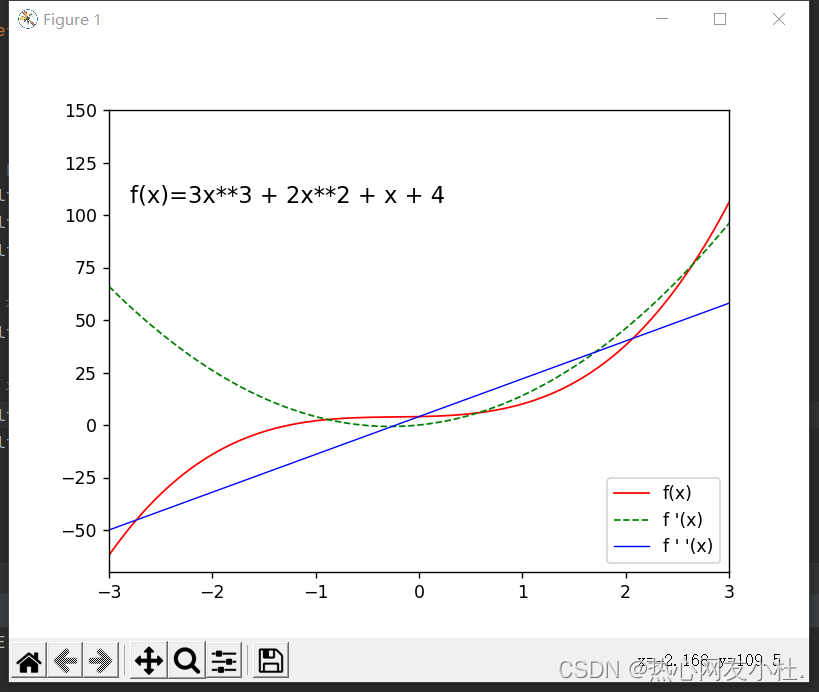那么我们同样可以画出其他函数的图像。例如正弦数(sinx)余弦函数(cosx),那么在画函数回像之前我们先要定义函数，即给出函数表达式。那么有小伙伴会问了：正、余弦函数应该如何定义？Y =对边／斜边？当然不是，而且这样写我们的程序也根本无法识别。这时我就要再次夸赞一下 我们 matplotlib库的方便处了。我们可以直接定义y = sin（x）或者y=cos（x），库里已经为我们定义好了函数，我们直接调用即可。代码如下：


import numpy as np
import matplotlib.pyplot as plt

x = np.linspace(-10, 10, 1000)
a = np.sin(x)
b = np.cos(x)
c = np.tan(x)

plt.figure(figsize=(8, 4))
plt.plot(x, a, label='$sin(x)$', color='green', linewidth=0.5)
plt.plot(x, b, label='$cos(x)$', color='red', linewidth=0.5)
plt.plot(x, c, label='$tan(x)$', color='blue', linewidth=0.5)

plt.xlabel('X')
plt.ylabel('Y')
plt.xlim(0, 10)
plt.ylim(-5, 5)
plt.legend()
plt.show()

此处定义函数时，只用a=np.sin（x）即可（cos、tan同理）。

plt.figure(figsize=(8, 4))

这里我们用到了一个新的函数plt.figure。简单介绍一下它的用法：

figure(num=None, figsize=None, dpi=None, facecolor=None, edgecolor=None, frameon=True)

num:图像编号或名称，数字为编号 ，字符串为名称
figsize:指定figure的宽和高，单位为英寸；
dpi参数指定绘图对象的分辨率，即每英寸多少个像素，缺省值为80      1英寸等于2.5cm,A4纸是 21*30cm的纸张
facecolor:背景颜色
edgecolor:边框颜色
frameon:是否显示边框

其他与我们之前的函数写法无异，接下来我们看函数图像：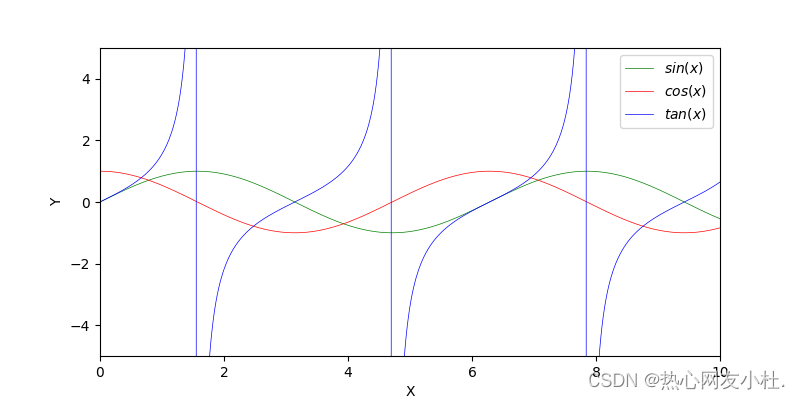接下来我们来介绍第三种函数：

记得我们高中的时候还学过一种很特殊的函数：椭圆。那么我们依旧可以拿matplotlib来画椭圆的函数图像。那么椭圆的函数图像我们应该如何定义呢？能否从库里直接调用？答案是不行的。库里并没有显示椭圆的函数，于是我们选择自定义函数的方法，来自定义一个椭圆方程。

import numpy as np

import matplotlib.pyplot as plt

plt.figure()

ax = plt.subplot()

x = np.linspace(0, np.pi, 100) # 在0到2pi之间，均匀产生100点的数组

# r不是半径

r = 2

ax.plot(r * np.cos(x) * np.cos(x), r * np.cos(x) * np.sin(x))

plt.show()


可以看到我们用到了一个新的函数：subplot。什么是subplot？

首先，它的调用是这样子的：subplot（numbRow ， numbCol ，plotNum ） or  subplot(numbRow numbCol plotNum)，对。看清楚，可以不用逗号分开直接写在一起也是对的；

解释一下这是啥玩意：

numbRow是plot图的行数；numbCol是plot图的列数；plotNum是指第几行第几列的第几幅图 ；

举个例子，如果是subplot （2 ，2 ，1），那么这个figure就是个2*2的矩阵图，也就是总共有4个图，1就代表了第一幅图

也可以写成subplot（221），这是没毛病的哈。是不是超级简单！

其次我们可以发现我们定义到了一个r=2但它不是半径，那么它是什么？其实它只是一个常数值，用来写椭圆参数函方程的常数值。其他都与我们之前讲的东西是一样的，所以我们来看函数图：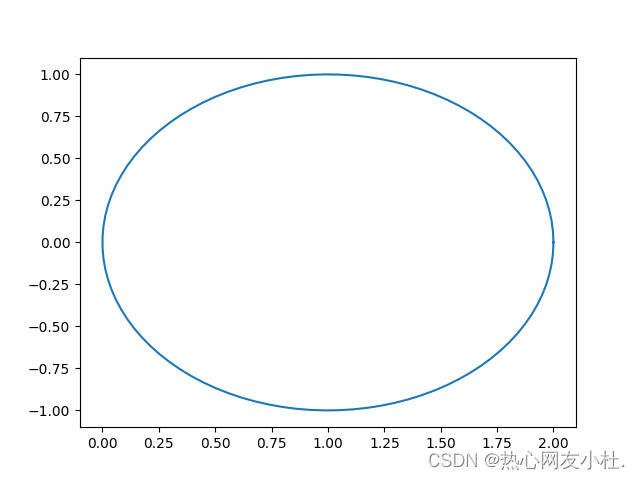以上就是我们今天介绍的全部内容了，之后将会继续更新有关知识，欢迎阅览~

展开全文python
• 1、用sympy import sympy from sympy import symbols, plot_implicit x, y = symbols('x y')#创建两个变量x和y ...#plot_implicit中的第一个参数即为所要绘制的方程，方程右边等于0 #在sympy.log(x,sympy.E)

1、用sympy

import sympy
from sympy import symbols, plot_implicit

x, y = symbols('x y')#创建两个变量x和y
plot_implicit(0.5*x-0.3*sympy.log(x,sympy.E)+
0.3*y-0.1*sympy.log(y,sympy.E) -1,(x,0,4),(y,0,4))
#plot_implicit中的第一个参数即为所要绘制的方程，方程右边等于0
#在sympy.log(x,sympy.E)中，sympy.E为自然对数的底

输出结果如下图所示：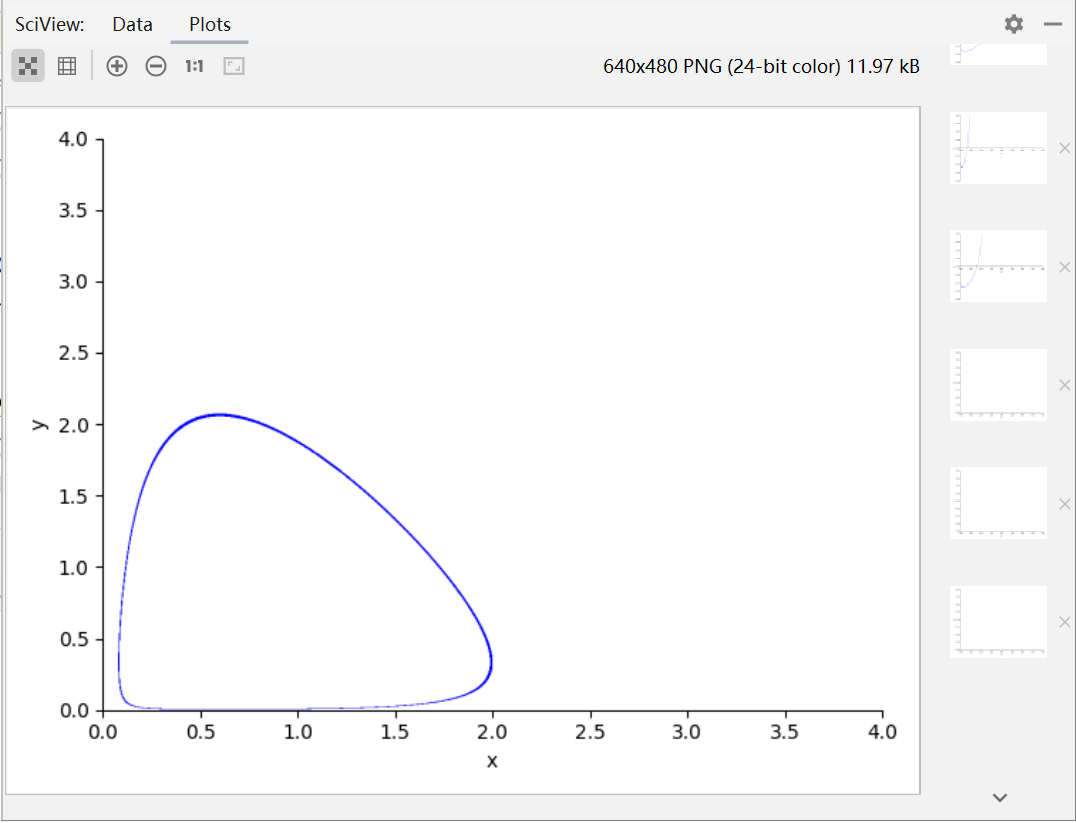2、用matplotlib

import matplotlib.pyplot as plt
import numpy as np
#作点
x=np.linspace(-1,4,1000)
y=np.linspace(-1,4,1000)

#构造网格
x,y=np.meshgrid(x,y)
z=0.5*x-0.3*np.log1p(x)+0.3*y-0.1*np.log1p(y)-1
#上式等号右边即为要绘制的方程，方程的右边等于0
#绘制等高线,8表示等高线数量加1
plt.contour(x,y,z,0)
plt.show()`

输出结果如下图所示：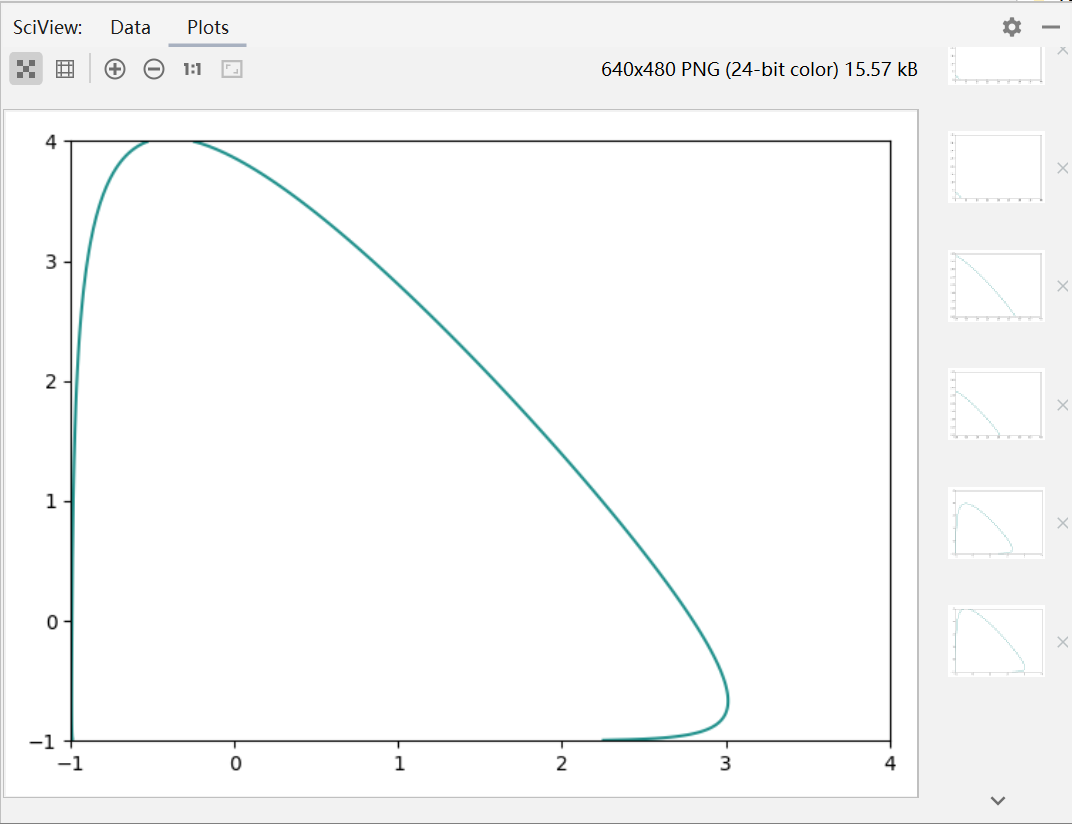展开全文python
• 安装的是anaconda, 其中包含了numpy, scipy, matplotlib 等科学计算包在终端打开anaconda中的python/home/qi/anaconda/bin/python>>>frommatplotlibimportpyplotaspl>>>importnumpyasnp>>&...

安装的是anaconda, 其中包含了numpy, scipy, matplotlib 等科学计算包

在终端打开anaconda中的python

/home/qi/anaconda/bin/python>>> from matplotlib import pyplot as pl

>>> import numpy as np

>>> from scipy import interpolate

>>> x = np.linspace(-10, 5, 100)

>>> y = -2*x**3 + 5*x**2 + 9

>>> pl.figure(figsize = (8, 4))

>>> pl.plot(x, y, color="blue", linewidth = 1.5)

[]

>>> pl.show()

pl.figure 设置绘图区大小

pl.plot    开始绘图, 并设置线条颜色, 以及线条宽度

pl.show 显示图像

显示图像如图参考:

http://zhan.renren.com/pythonlover?gid=3602888498028996903

展开全文• 1.前言 matplotlib 是 python 最著名的绘图库，它提供了一整套和 matlab 相似的命令 ...这是要实现的效果图，利用 Matplotlib 绘制函数 y = x^2^ 的图像。 1.1 代码实现 import matplotlib.pyplot as plt imporpython
• Pyplot 包含一系列绘图函数的相关函数，每个函数会对当前的图像进行一些修改。本文通过绘制三角函数cos()来对其中一些重要函数进行讲解。 1、绘图 （1）导入所需库 import numpy as np import matplotlib.pyplot as ...python
• # 导入相关的包import numpy as npimport matplotlib.pyplot as pltimport matplotlib as mpl#%%配置全局绘图参数#设置字体大小mpl.rcParams["font.size"] = 14#设置画布大小mpl.rcParams["figure.figsize"] = (12,7...
• 一、matplotlib绘制散点图 # coding=utf-8 import matplotlib.pyplot as plt years = [2009, 2010, 2011, 2012, 2013, 2014, 2015, 2016, 2017, 2018, 2019] turnovers = [0.5, 9.36, 52, 191, 350, 571, 912, 1027...
• 这篇经验告诉你，如何利用python的matplotlib模块三角函数图像，包括正弦、余弦、正切、余切。工具/原料windows系统电脑一台python软件，并安装matplotlib模块、numpy模块spyder编辑器方法/步骤1第一步，点击键盘 ...
• matplotlib 是 python 最著名的绘图库，它提供了一整套和 matlab 相似的命令 API，十分适合交互式进行绘图。它的文档相当完备，并且 ...这是要实现的效果图，利用 Matplotlib 绘制函数 y = x^2^ 的图像。image代...
• matplotlib是Python最著名的绘图库，本文给大家分享了利用matplotlib+numpy绘制多种绘图的方法实例，其中包括填充图、散点图(scatter plots)、. 条形图(bar plots)、等高线图(contour plots)、 点阵图和３Ｄ图，需要...
• 本文实例讲述了Python实现在tkinter中使用matplotlib绘制图形的方法。分享给大家供大家参考，具体如下： 一. 代码： # coding=utf-8 import sys import Tkinter as Tk import matplotlib from numpy import arange,...
• 微积分的极限一章上 y=sin(x)/x 在x趋近为0的极限为1，而且还有几何很直观的证明，这里就用pydroid画一下它的函数图像，曲线还是挺好看的 import matplotlib.pyplot as plt import numpy as np x = np.linspace(-16...
• 绘制函数图像4.散点图和柱状图的绘制 一、什么是Matplotlib 是专门用于开发2D图表(包括3D图表) 使用起来及其简单 以渐进、交互式方式实现数据可视化 二、Matplotlib图像结构 三层结构 三、绘图 1.基础绘图 ...机器学习 python
• 使用python中的第三方库matplotlib绘制正余弦函数图形，并标注x，y轴以及标题等 ，绘制方法与matlab类似，可以说这里的matplotlib库便是Python中的matlab 程序代码如下： #绘制正余弦函数图像 import numpy as np...python pycharm
• 实训1 分析1996-2015年...import matplotlib.pyplot as plt #扩展语言，使中文可以使用 plt.rcParams["font.sans-serif"]="SimHei" plt.rcParams["axes.unicode_minus"]=False #读取数据 t=np.load('G:/大数据实验...大数据 python
• 使用pytorch实现一个简单的线性模型，并调用matplotlib输出模型图像。 （本菜鸡注释真的多...） import torch import numpy as np ...from mpl_toolkits.mplot3d import Axes3D #绘制3D坐标的函数 x_.python
• Matplotlib绘制各种图片前言绘制RGB图像绘制灰度图绘制PIL格式图片绘制opencv格式的图片其他技巧 前言 在使用Jupyter Notebook时，我们希望利用Matplotlib的inline模式直接在网页上绘制出图片。本文将介绍使用...python opencv
•直方图
• 本文主要介绍如何通过pyplot来绘制函数图。主要绘制函数如下： – 一元一次函数 – 一元二次函数 – 指数函数 – 自然对数函数 – 正弦函数 一元一次函数 代码 import matplotlib.pyplot as plt import numpy as ...
• import matplotlib.pyplot as plt classes = ['猫','猴子','狗','兔子'] confusion_matrix = np.array([(666,7,43,18),(6,822,0,35),(24,1,668,1),(7,1,1,339)],dtype=np.float64) proportion=[] for i in ...python 数据挖掘 计算机视觉
• python实现绘制二次函数图像 python实现绘制三维函数图像 python实现绘制二次函数图像 import matplotlib.pyplot as plt import numpy as np import math x = np.linspace(-10, 10, 10) #分别代表最小，最大，...python 开发语言 后端
• Matplotlib利用指数函数的向量及其逆向向量绘图实例python
• 运行环境 : python 3.6.0 用 Matplotlib 的 scatter 方法绘制散点图 核心代码 : # -*- encoding: utf-8 ...# @Function : 本代码为利用 Matplotlib 库画正余弦函数 import numpy as np from matplotlib impor...Python
• 本题以画函数f(x) = xsin(10pi*x) + 2.0图像为例。 import numpy as np # 1000是设置-1到2之间的数据量 x = np.linspace(-1, 2, 1000) y = x*np.sin(10*np.pi*x) + 2.0 import matplotlib.pyplot as plt plt.plot...python
• 1、定义一个画圆的函数 import numpy as np import matplotlib.pyplot as plt def plot_circle(center=(3, 3),r=2): x = np.linspace(center - r, center + r, 5000) y1 = np.sqrt(r**2 - (x-center)**2...
• ## Matplotlib绘制动图

千次阅读 多人点赞 2020-09-21 16:13:22...